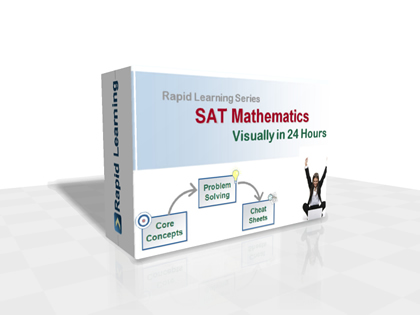How to Learn in 24 Hours?The Rapid Learning Movie

 Need Help? M-F: 9am-5pm (PST): Toll-Free: (877) RAPID-10 or 1-877-727-4310 24/7 Online Technical Support: The Rapid Support Center Secure Online Order:Need Proof? Testimonials by Our Users

 Rapid Learning Courses: MCAT in 24 Hours (2019-20) USMLE in 24 Hours (Boards) Chemistry in 24 Hours Biology in 24 Hours Physics in 24 Hours Mathematics in 24 Hours Psychology in 24 Hours SAT in 24 Hours ACT in 24 Hours AP in 24 Hours CLEP in 24 Hours DAT in 24 Hours (Dental) OAT in 24 Hours (Optometry) PCAT in 24 Hours (Pharmacy) Nursing Entrance Exams Certification in 24 Hours eBook - Survival Kits Audiobooks (MP3)

 Tell-A-Friend: Have friends taking science and math courses too? Tell them about our rapid learning system.Home »  SAT » SAT MathCourse Preview

The SAT Math Rapid Learning Series includes:

1. 24 Core Concept Tutorials (Flash Movies)
Concept maps, easy introduction to the topic, concept illustration with annotations and animations, sample problems explained with step-wise strategies and topic summary.
2. 24 Problem-Solving Drills (Flash Games)
Feedback based Quizzes, concept-based problems, summary reviews on all problems and scoring system to track performance.
3. 24 Super Review Cheat Sheets (PDF Printables)
One cheat sheet per topic, key concepts in a single-sheet, at-a-glance review of each topic and both printable and laminatible Ideal for exam prep quick review.
4. 24 Quick-Read Chapter Mini-Books (PDF Printables)
24 Slide-by-slide printable eBooks for each chapter, as the study companion of the visual tutorials.
5. 24 Learning-on-the-Go AudioBooks (MP3)
24 mp3 audio tutorials for 24 chapters, perfect supplement to the visual tutorials.

RL531: SAT Math Visually in 24 Hours

SAT (SAT Reasoning Test) is a standardized test for high school achievement and college admissions, administered by the College Board. The required math topics could be overwhelming to study by self reading in absence of classroom instructions. Rapid Learning overcomes this learning gap by offering visual lectures developed and narrated by math and science educators. Let our experts guide you through your study by following our signature Rapid Learning System - Visual Tutorials + Problem Drills + Cheat Sheets.

This visual course provides the comprehensive coverage of the math required topics by the SAT, with one-to-one match with the official required topics. Study the most relevant content and study them powerfully with Rapid Learning.

Core Unit #1 – Numbers and Operations

• Tutorial 01: Basic Arithmetic and Numbers
• Tutorial 02: Properties of Integers and Algebraic Expressions
• Tutorial 03: Exponents and Radical (Square and Square Root)
• Tutorial 04: Fractions and Rational Numbers
• Tutorial 05: Ratio, Proportion and Percent
• Tutorial 06: Sets - Union, Intersection and Elements

Core Unit #2 – Algebra and Functions

• Tutorial 07: Basic Algebra
• Tutorial 08: Simple Equations
• Tutorial 09: Multi-Step Equations and Direct Variation
• Tutorial 10: Inequalities
• Tutorial 11: Absolute Value: Functions and Inequalities
• Tutorial 12: Systems of Linear Equations and Inequalities
• Tutorial 13: Rational Equations and Inequalities
• Tutorial 14: Basic Graphs and Data Representation
• Tutorial 15: Functions, Graphs and Properties
• Tutorial 16: Linear Functions, Equations and Graphs
• Tutorial 17: Quadratic Functions, Equations and Graphs
• Tutorial 18: Applied Math and Word Problems in Algbera

Core Unit #3 – 2D amd 3D Geometry

• Tutorial 19: Plane Geometry
• Tutorial 20: Geometric Perimeters and Areas
• Tutorial 21: Solid Geometry: Surface and Volume
• Tutorial 22: Coordinate Geometry

Core Unit #4 – Data Analysis, Statistics and Probability

• Tutorial 23: Data Interpretation and Statistics
• Tutorial 24: Elementary Probability and Geometric Probability

Course Preview

Disclaimer: SAT is a registered trademark of the College Board, which does not endorse, nor is affiliated in any way with the Rapid Learning courses.Home »  SAT » SAT Math